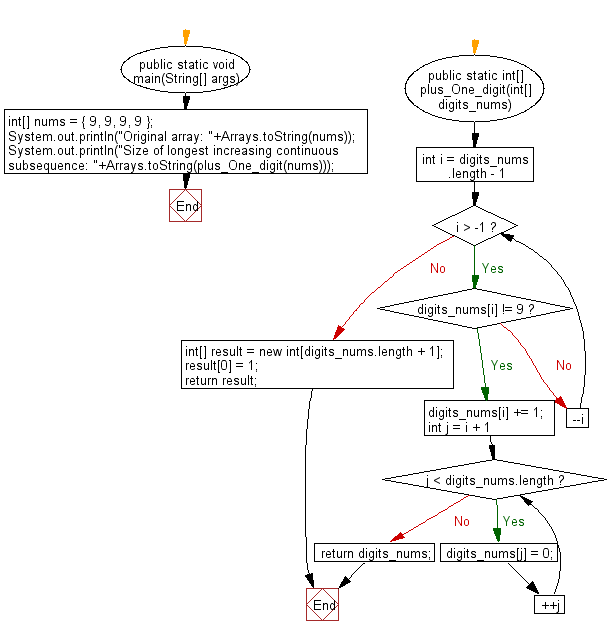﻿ Java programming exercises: Plus one to the number of a specified positive numbers represented as an array of digits - w3resource# Java Exercises: Plus one to the number of a specified positive numbers represented as an array of digits

## Java Basic: Exercise-179 with Solution

Write a Java program to plus one to the number of a given positive numbers represented as an array of digits.

Sample array: [9, 9, 9, 9] which represents 9999
Output: [1, 0, 0, 0, 0].

Sample Solution:

Java Code:

``````import java.util.*;
public class Solution {
public static void main(String[] args) {
int[] nums = {9,9,9,9};
System.out.println("Original array: " + Arrays.toString(nums));
System.out.println("Array of digits: " + Arrays.toString(plus_One_digit(nums)));
}
public static int[] plus_One_digit(int[] digits_nums) {
for (int i = digits_nums.length - 1; i > -1; --i) {
if (digits_nums[i] != 9) {
digits_nums[i] += 1;
for (int j = i + 1; j < digits_nums.length; ++j) {
digits_nums[j] = 0;
}
return digits_nums;
}
}
int[] result = new int[digits_nums.length + 1];
result = 1;
return result;
}
}
```
```

Sample Output:

```Original array: [9, 9, 9, 9]
Array of digits: [1, 0, 0, 0, 0]
```

Flowchart:Java Code Editor:

What is the difficulty level of this exercise?

﻿

## Java: Tips of the Day

Parsing dates:

```import java.io.*;
import java.util.*;
import java.text.*;

String s = "2001/09/23 14:39";

SimpleDateFormat formatter = new SimpleDateFormat ("yyyy/MM/dd H:mm");
Date d = formatter.parse(s, new ParsePosition(0));
```